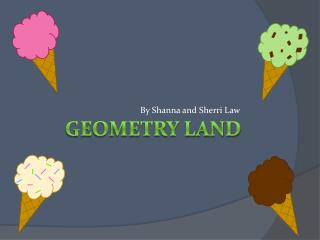DownloadDownload PresentationGeometry land

# Geometry land

Download Presentation## Geometry land

- - - - - - - - - - - - - - - - - - - - - - - - - - - E N D - - - - - - - - - - - - - - - - - - - - - - - - - - -
##### Presentation Transcript

1. Geometry land By Shanna and Sherri Law

2. Finish Questions

3. Question 1 Question 2 Question 3 Question 4 Question 5 Question 6 Question 7 Question 8 Question 9 Question 10 Question 11 Question 12 Question 13 Question 14 Question 15 Question 16 Question 17 Question 18 Question 19 Question 20 Question 21 Question 22 Question 23 Question 24 Question 25 Question 26 Question 27 Question 28 Question 29 Question 30 Question 31 Question 32 Question 33 Question 34 Question 35 Question 36 Question 37 Question 38 Question 39 Question 40 Question 41 Question 42 Question 43 Question 44 Question 45 Question 46 Question 47 Question 48 Question 49 Question 50 Question 51 Question 52 Question 53 Question 54 Question 55 Question 56 Question 57 Question 58 Question 59 Question 60

4. Find the next object in the pattern

5. Game Board

6. What is a counterexample?

7. An example that proves a conjecture false. Game Board

8. The ruler postulate states that the _____ on a line can be ______ one to one with real numbers.

9. points; matched Game Board

10. Define segment bisector

11. A segment, line, ray, or plane that intersects a segment at its midpoint Game Board

12. What is the Distance Formula?

13. AB = √(x2-x1)2+(y2-y1) 2 Game Board

14. AB=AD means that the lengths are equal, and AB AD means that the segments are congruent ~ = Game Board

15. Define collinear points.

16. Points that lie on the same line. Game Board

17. CE=14 Game Board

18. What is the midpoint of a segment?

19. A point that divides, or bisects, the segment into two congruent segments Game Board

20. What are vertical angles?

21. even Game Board

22. What has no dimension and is often represented by a dot?

23. a point Game Board

24. What are rules that are accepted without proof?

25. postulates Game Board

26. Name the postulate. If B is between A and C, then AB+BC=AC. If AB+BC=AC, then B is between A and C.

27. Segment Addition Postulate Game Board

28. What is inductive reasoning?

29. Making conjectures and looking for patterns Game Board

30. Name all the points that are NOT coplanar with point A H G C B E F A D

31. G Game Board

32. What is the Distance Formula used for?

33. To find the distance between two points in a coordinate plane. Game Board

34. CA and CB are opposite rays if _________.

35. C lies between A and B Game Board

36. What is a conjecture?

37. What formula is this: If A(x1, y1) and B(x2, y2) are points in a coordinate plane, then the midpoint of AB has coordinates x1+x2 y1+y2 ( ) ____ ____ , 2 2

38. The Midpoint Formula Game Board

39. The sides of an angle are made of _______.

40. rays Game Board

41. What is this: Every even number greater than 2 can be written as the sum of two primes

42. Goldbach’s Conjecture Game Board

43. What are complementary angles?

44. two angles whose measures add up to 90° Game Board